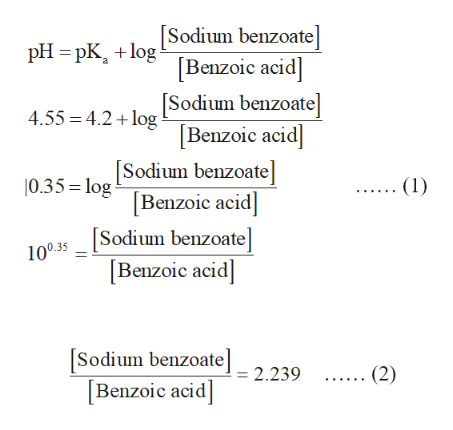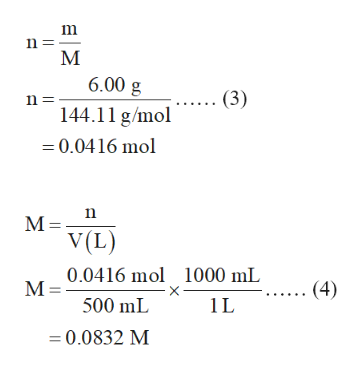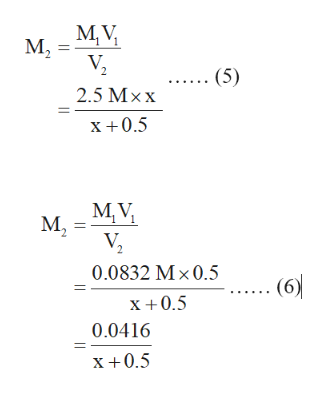# How many milliliters of 2.5M benzoic acid need to be added to 500. mL of a solution already contains 6.00 grams of sodium benzoate, if you want the final pH to be 4.55? Ka for benzoic acid =6.28x10^-5 (B)if you add 16 grams of sodium benzoate to 4.55 grams of benzoic acid to a 1.00 liter container of water, what is the pH of this solution? (c)how many grams of potassium nitrite need to be added to 250.0 mL of a 0.1500 M HNO2 solution to produce a final buffer at pH=3.65? Ka for HNO2=4.5x10^-4

Question
182 views

How many milliliters of 2.5M benzoic acid need to be added to 500. mL of a solution already contains 6.00 grams of sodium benzoate, if you want the final pH to be 4.55? Ka for benzoic acid =6.28x10^-5

(B)if you add 16 grams of sodium benzoate to 4.55 grams of benzoic acid to a 1.00 liter container of water, what is the pH of this solution?

(c)how many grams of potassium nitrite need to be added to 250.0 mL of a 0.1500 M HNO2 solution to produce a final buffer at pH=3.65? Ka for HNO2=4.5x10^-4

check_circle

Step 1

The value of pKa is calculated below.

pKa = -logKa

= - log (6.28 × 10-5)

= 4.2

The ratio of sodium benzoate to benzoic acid is calculated in equation (1) with the help of Henderson-Hasselbalch equation.

…… (1)

On solving it further as shown in equation (2),help_outlineImage TranscriptioncloseSodium benzoate Benzoic acid] [Sodium benzoate] [Benzoic acid pH pKlog 4.55 4.2 log 0.35 Sodium benzoate (1) [Benzoic acid] Sodium benzoate] Benzoic acid 100.35 Sodium benzoate] [Benzoic acid] ...... (2) 2.239 fullscreen
Step 2

The molar mass of sodium benzoate is 144.11 g/mol. The number of moles (n) of sodium benzoate is calculated in equation (3).

…… (3)

The molarity of sodium benzoate is calculated in equation (4) by substituting 500 mL forV and 0.0416 mol for n.help_outlineImage TranscriptioncloseМ 6.00 g .. (3) n 144.11 g/mol =0.0416 mol М-. V(L) 0.0416 mol М- 1000 mL (4) 500 mL 1L =0.0832 M fullscreen
Step 3

The initial concentration (M1) of Benzoic acid is 2.5 M. The volume (V2) after the addition of two solution becomes x L + 0.5 L. Thus, the final concentration (M2) of Benzoic acid is given in equation (5).

The initial concentration (M1) of sodium benzoa...help_outlineImage TranscriptioncloseM,V М, (5) 2.5 Мxx х +0.5 M,V М, 0.0832 Мx0.5 (6) х +0.5 0.0416 х +0.5 fullscreen

### Want to see the full answer?

See Solution

#### Want to see this answer and more?

Solutions are written by subject experts who are available 24/7. Questions are typically answered within 1 hour.*

See Solution
*Response times may vary by subject and question.
Tagged in

### Equilibrium Concepts